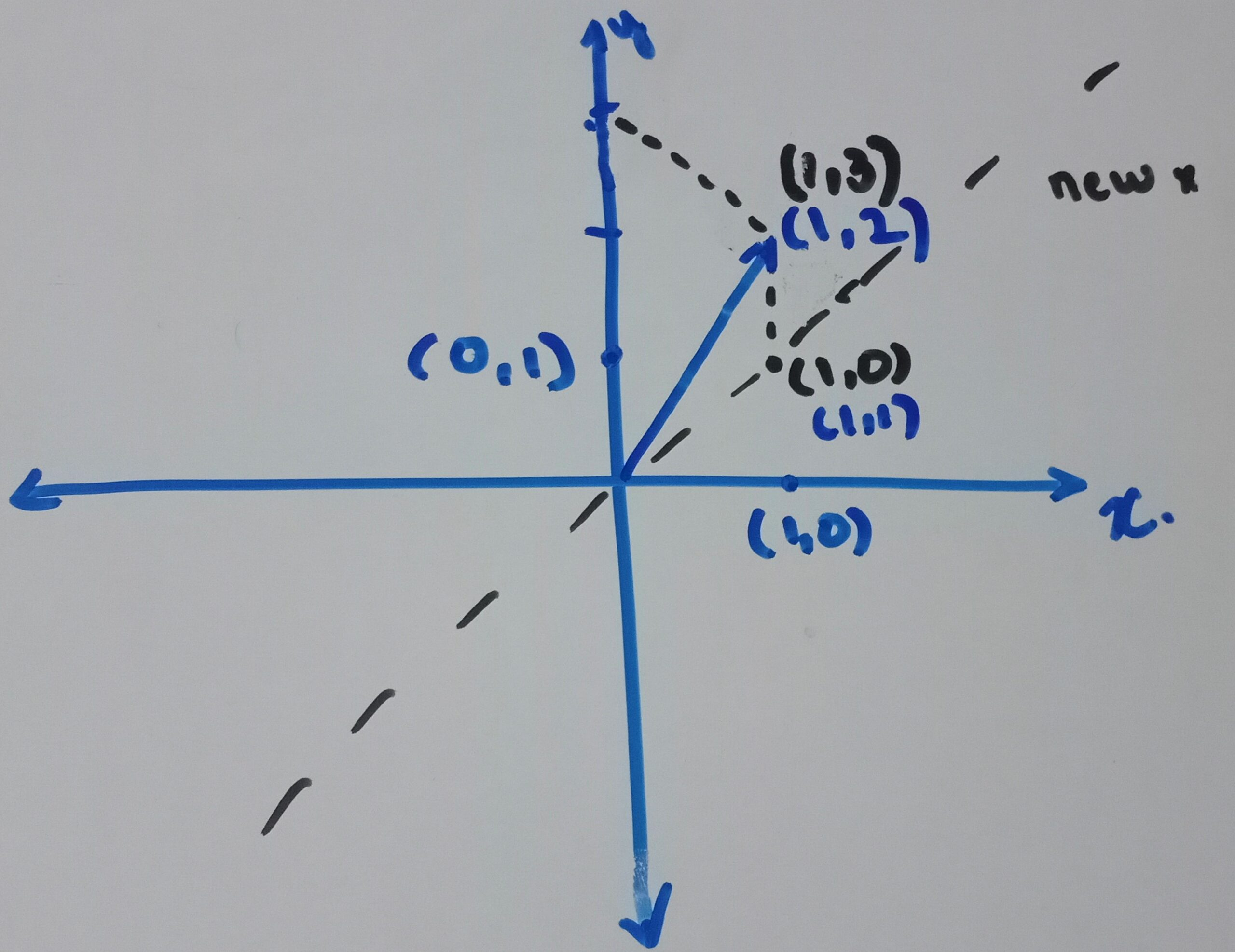# Matrices (R)

## Matrix multiplication¶

Matrix multiplication is an operation that produces a matrix from two matrices. The number of columns in the first matrix must be equal to the number of rows in the second matrix. The resulting matrix, known as the matrix product, has the number of rows of the first and the number of columns of the second matrix. The product of matrices $A$ and $B$ is then denoted simply as $AB$. - Wikipedia

Matrix multiplication is a linear transformation. What does that mean?

Consider the matrices

$A=\left[\begin{matrix}1&0\\1&1\\\end{matrix}\right],\ B\ =\ \left[\begin{matrix}1\\2\\\end{matrix}\right]$

$A\times b\ =\ 1\times\left[\begin{matrix}1\\1\\\end{matrix}\right]+2\times\left[\begin{matrix}0\\1\\\end{matrix}\right]=\left[\begin{matrix}1\\3\\\end{matrix}\right]$

What does this mean? This means that the first row of b is multiplied by the first columnin A, and the second row in b is multiplied by the second column in A. Visually it means that the points [1,0] and [0,1] are shifted to [1,1] and [0,1] in the x-y coordinate system. The new x-axis is the line which runs from [0,0] to [1,1] with unit amount at [1,1]. The new y-axis remains the same at [0,1]. After multiplying, the result is the coordinates of the vector b in the new coordinate system. Refer to this video for a more visual explanation.A = matrix(c(1,0, 1, 1), nrow = 2, byrow = T)
b = matrix(c(1,2), nrow = 2, byrow = T)
A %*% b

##      [,1]
## [1,]    1
## [2,]    3


The identity matrix is a square matrix with all diagonal elements as 1 and rest as 0. We can see that in the identity matrix, the axes are not shifted. Therefore the multiplication of any matrix with I gives itself. The identity matrix in 2x2 is

$I = \left[\begin{matrix}1&0\\0&1\\\end{matrix}\right]$

## Transpose of a matrix¶

The transpose of a matrix has the rows and columns transposed or interchanged.

t(A)

##      [,1] [,2]
## [1,]    1    1
## [2,]    0    1

t(b)

##      [,1] [,2]
## [1,]    1    2


The inner product of two matrices can be written as a product of one of the transposes. $U.V = U^T \times V$ The transpose of a product $(AB)^T = B^T A^T$

## Range, Rank and fundamental spaces¶

The range of a matrix is the set of vectors that can be obtained as a linear combination of the columns of A. We can call the range of the matrix as the span of all the column vectors. The dimension of the subspace created by this linear combination is the rank of the matrix. Consider two examples:

$A=\left[\begin{matrix}1&0\\1&1\\\end{matrix}\right], C=\left[\begin{matrix}1&2\\0&0\\\end{matrix}\right]$

$A\times x$ spans the whole $R^2$ space as the two column vectors $a_1 = [1,1]$ and $a_2=[0,1]$ are linearly independent.

On the other hand, $C\times x$ gives us a straight line on the x-axis.

$\left[\begin{matrix}1&2\\0&0\\\end{matrix}\right] \times \left[\begin{matrix}x_1\\x_2\\\end{matrix}\right] = \left[\begin{matrix}x_1+2x_2\\0\\\end{matrix}\right]$

The matrix C spans a straight line only. The range for matrix c are the vectors on the x-axis, and the rank is one, which is the dimension of a line.

The column rank of $A \in R^{mxn}$ is the maximum number of linearly independent columns, and the row rank is the maximum number of linearly independent rows. The column rank is the size of the basis of $A$, and the row rank is the size of the basis for $A^T$. Refer to this video for a more visual explanation.

library(Matrix)
rankMatrix(A)

##  2

C = matrix(c(1,0, 2, 0), nrow = 2, byrow = T)
rankMatrix(C)

##  1


Vectors that output zero vectors when multiplied with matrix A are calles the Nullspace. The dimension of the null space is called the null rank. For matrix $A$, only the null vector $x=[0,0]$ is the null space, and therefore the null rank is 0. For the matrix C, any vector of form $x_1+2x_2 = 0$ will form the null space of the matrix. The null rank for C is one as the null space is a line. Notice that the rank of null space + column rank of the matrix = number of columns.

## Determinants¶

In $R^2$, the determinant is the area of the parallelogram that is mapped by the unit square after multiplying with the matrix. Similarly, in $R^3$, it is the area of the parallelotope that is mapped by the unit cube after multiplying with the matrix. Refer to this video for a more visual explanation.

det(A)

##  1

det(C)

##  0